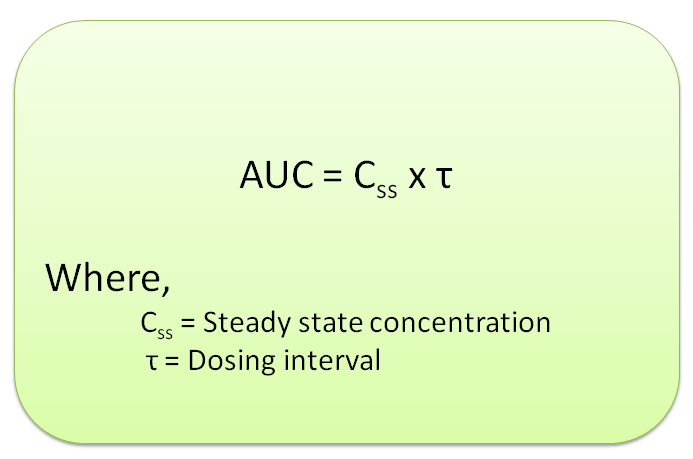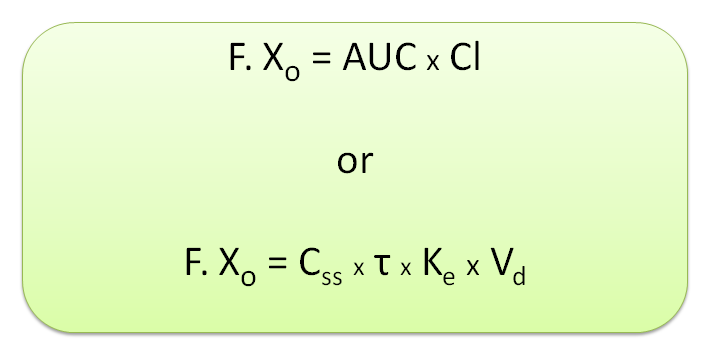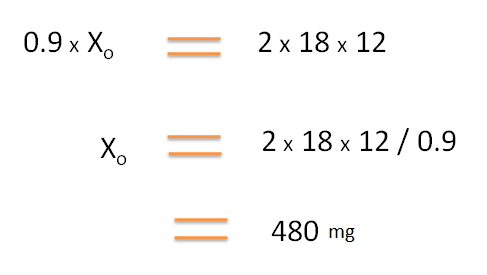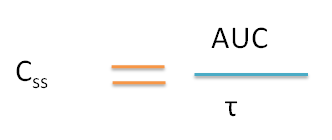1. Home
2. â€º
3. Blog
4. â€º

# Biopharmaceutical calculations in sustain release products

by egpat       08-06-2017

Sustained release products release the drug slowly so that drug levels in the body can be maintained at required levels. When these products are given at certain dosing intervals a steady state concentration can be achieved. Suppose a sustained release product is given at fixed dosing intervals to achieve a steady state concentration of Css, the total amount of the drug in the body can be obtained by multiplication of average steady state concentration with dose interval. As AUC indicates total amount of drug absorbed into the systemic circulation, the following equation can be obtained.Since AUC is related with dose, we can draw an expanded equation as follows.Now let's see few working examples on sustained release products.

Working example 1: Calculation of dose

How much dose of a sustained release preparation is required at every 12 hr to attain a steady state plasma concentration of 2 mg/L. The clearance of the drug is 18 L/hr and 90% of drug is absorbed.

Solution:

Data given:

Clearance=18 L/hr

Dosing interval, tau=12 hr

Fraction of dose absorbed, F=90%=0.9

What is required

Dose Xo=?

By applying above equation,Working example 2: Calculation of Css

A 600 mg of sustained release product was given for every 12 hr. If the clearance of the drug is 25 L/hr, the average steady state plasma concentration achieved is ?

Data given:

Dose Xo=600mg

Clearance=25 L/hr

Dosing interval, tau=12 hr

Fraction of dose absorbed is not given hence assumed to be equal to 1.Working example 3: Calculation of dose

How much dose of a sustained release preparation is required at every 12 hr to attain a steady state plasma concentration of 5 mg/L. The clearance of the drug is 10 L/hr

Data given:

Dose Xo=?

Clearance=10 L/hr

Dosing interval, tau=12 hr

Fraction of dose absorbed is not given hence assumed to be equal to 1.Working example 4: Calculation of Css

A 300-mg dose of a drug is given every 5 hours until a steady-state level is reached. At steady state, AUC for one dosing interval is 50 (mg/L) x hour. What is the average concentration over that dosing interval?

Data given:

AUC=50 (mg/L) ´ hour

Dosing interval, tau=5 hr

Dose, Xo=300 mg

What is required

Here we can use the following equation.Hence average steady state concentration, Css=50/5=10

Note: When AUC and dosing interval are known, Css can be directly calculated and dose is not required.

Working example 5: Calculation of Css

A patient was given 500 mg of dose at every 8 hr until a steady state is reached. If the clearance of the drug is 5 L/hr, the average steady state concentration is ?

Data given:

Dose Xo=500 mg

Clearance=5 L/hr

Dosing interval, tau=8 hr

Fraction of dose absorbed is not given hence assumed to be equal to 1.

What is requiredWorking example 6: Calculation of dose

How much dose is required for a sustained release product with volume of distribution as 40L to achieve a steady state concentration of when given at every 8 hours. The elimination rate constant of the drug is 0.5 hr-1.

Data given :

Average steady state concentration, Css=5 mg/L

Dosing interval=8 hr

Elimination rate constant, Ke=0.5 hr-1

Volume of distribution, Vd=20 L

Fraction of dose absorbed=1

What is required

Dose=?